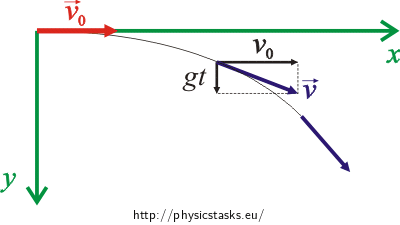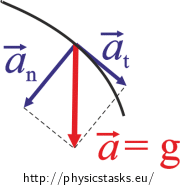## Bullet in Vacuum

A bullet was shot horizontally in a vacuum with speed v0 (speed refers to the magnitude of velocity). Determine the dependencies of speed v and magnitudes of tangential acceleration at and normal acceleration an on time t.

Determine the behaviour of the radius of curvature ρ of the trajectory depending on time t.

• #### Hint 1: Free body diagram

Draw a free body diagram illustrating the situation and mark coordinate axes x and y into it.

Draw the vector of the initial velocity of the bullet $$\vec{v}_0$$ and its velocity $$\vec{v}$$ at an arbitrary point of its motion trajectory as well as its components in directions of the coordinate axes.

• #### Hint 2: Behaviour of speed

Think about what kinds of motion the bullet follows in directions of the coordinate axes.

First, express the components of the velocity of the bullet vx, vy. The behaviour of the speed of the bullet can be easily determined from them.

• #### Hint 3: Magnitude of tangential acceleration

Use the knowledge of the speed of the bullet from the previous hint. How can you use it to express the magnitude of the tangential acceleration of the bullet?

• #### Hint 4: Magnitude of normal acceleration

To determine the magnitude of the normal (centripedal) acceleration of the bullet you need the magnitude of its tangential acceleration (known from the previous hint) as well as the magnitude of its net acceleration, which you can obtain from the components of acceleration ax, ay in directions of the coordinate axes.

What relation holds between the net, tangential and normal accelerations of the bullet?

• #### Hint 5: Radius of the trajectory curvature

The trajectory of the bullet is drawn in the free body diagram.

What is the relation between the radius of curvature of the trajectory, the speed and the magnitude of the normal acceleration of the bullet?

• #### OVERALL SOLUTION

A free body diagram illustrating the situation:In the direction of the x axis: a uniform linear motion with speed v0.

In the direction of the y axis: a free fall

Components of velocity (in directions of the x axis and the y axis):

$v_\mathrm{x}\,=\,v_0\,,$ $v_\mathrm{y}\,=\,gt\,.$

Behaviour of speed

$v(t) \,=\, \sqrt{v_\mathrm{x}^{2}\,+\,v_\mathrm{y}^{2}}\,=\,\sqrt{v_0^{2}\,+\,g^{2}t^{2}}\,.$

Magnitude of the tangential acceleration:

We obtain the magnitude of tangential acceleration by differentiating speed with respect to time:

$a_\mathrm{t}\,=\,\frac{\mathrm{d}v}{\mathrm{d}t}\,=\,\frac{\mathrm{d}\left(\sqrt{v_0^{2}\,+\,g^{2}t^{2}}\right)}{\mathrm{d}t}\,=\,\frac{g^{2}t}{ \sqrt{v_0^{2}\,+\,g^{2}t^{2}}}\,.$

Magnitude of the net acceleration

We obtain the magnitude of normal acceleration from relation:

$a\,=\,\sqrt{a_\mathrm{t}^{2}+ a_\mathrm{n}^{2}}\,.$

From here:

$a_\mathrm{n}\,=\,\sqrt{a^{2}-a_\mathrm{t}^{2}}\,,$

Components of acceleration:

$a_\mathrm{x}\,=\,\frac{\mathrm{d}v_\mathrm{x}}{dt}\,=\,\frac{\mathrm{d }\left(v_0\right)}{\mathrm{d}t} \,=\, 0\,,$ $a_\mathrm{y}\,=\,\frac{\mathrm{d}v_\mathrm{y}}{\mathrm{d}t}\,=\, \frac{\mathrm{d}\left(gt\right)}{\mathrm{d}t} \,=\, g\,,$$a \,=\, \sqrt{a_\mathrm{x}^{2}+ a_\mathrm{y}^{2}} \,=\, g\,.$ $a_\mathrm{n}\,=\,\sqrt{g^{2}-\frac{g^{4}t^{2}}{v_0^{2}+g^{2}t^{2}}} \,=\, \sqrt{\frac{g^{2}v_0^{2}}{v_0^{2}+g^{2}t^{2}}}\,,$ $a_\mathrm{n}\,=\,\frac{gv_0}{\sqrt{v_0^{2}+g^{2}t^{2}}}\, .$

For the magnitude of normal acceleration it holds that:

$a_\mathrm{n} \,=\,\frac{v^{2}}{\rho}\,.$

It therefore holds for the radius of curvature that:

$\rho\,=\,\frac{v^{2}}{a_\mathrm{n}}\,,$

where

$v \,=\, \sqrt{v_0^{2}+g^{2}t^{2}}\,,$ $a_\mathrm{n}\,=\,\frac{gv_0}{\sqrt{v_0^{2}+g^{2}t^{2}}}\,.$ $\rho(t)\,=\,\frac{ (v_0^{2}+g^{2}t^{2})\sqrt{v_0^{2}+g^{2}t^{2}}}{gv_0}\,=\,\frac{\sqrt{( v_0^{2}+g^{2}t^{2})^{3}}}{gv_0}\,.$

The behaviour of the speed of the bullet is

$v(t) \,=\, \sqrt{v_0^{2}+g^{2}t^{2}}\,.$

The magnitude of the tangential acceleration of the bullet is

$a_\mathrm{t}(t) \,=\, \frac{g^{2}t}{ \sqrt{v_0^{2}+g^{2}t^{2}}}\,.$

The magnitude of the normal acceleration of the bullet is

$a_\mathrm{n}(t) \,=\, \frac{gv_0}{\sqrt{v_0^{2}+g^{2}t^{2}}}\,.$

It holds for the radius of the trajectory curvature of the bullet that

$\rho(t) \,=\, \frac{\sqrt{( v_0^{2}+g^{2}t^{2})^{3}}}{v_0g}\,.$×Original source: Mandíková, D., Rojko, M.: Soubor úloh z mechaniky pro studium učitelství. I. část. Interní materiál, MFF UK, Praha 1994
Zpracováno v diplomové práci Jany Moltašové (2011).